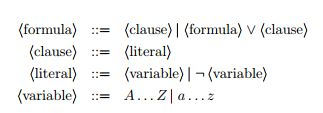### Описание

Ограничение по времени: 3000 ms
Ограничение по памяти: 512 Mb

### Задача: Boolean Satisfiability

Boolean satisfiability problem (SAT) is known to be a very hard problem in computer science. In this problem you are given a Boolean formula, and you need to find out if the variables of a given formula can be consistently replaced by the values true or false in such a way that the formula evaluates to true. SAT is known to be NP-complete problem. Moreover, it is NP-complete even in case of 3-CNF formula (3-SAT). However, for example, SAT problem for 2-CNF formulae (2-SAT) is in P.

#SAT is the extension of SAT problem. In this problem you need to check if it is possible, and count the number of ways to assign values to variables. This problem is known to be #P-complete even for 2-CNF formulae. We ask you to solve #1-DNF-SAT, which is #SAT problem for 1-DNF formulae.
You are given a Boolean formula in 1-DNF form. It means that it is a disjunction (logical or) of one or more clauses, each clause is exactly one literal, each literal is either variable or its negation (logical not). Formally:Your task is to find the number of ways to replace all variables with values true and false (all occurrences of the same variable should be replaced with same value), such that the formula evaluates to true.

Input
The only line of the input file contains a logical formula in 1-DNF form (not longer than 1000 symbols). Logical operations are represented by ‘|’ (disjunction) and ‘~’ (negation). The variables are ‘A’ . . . ‘Z’ and ‘a’ . . . ‘z’ (uppercase and lowercase letters are different variables). The formula contains neither spaces nor other characters not mentioned in the grammar.

Output
Output a single integer — the answer for #SAT problem for the given formula

 Input Output a 1 B|~B 2 c|~C 3 i|c|p|c 7

Прикрепите файл с исходным кодом программы:

или введите исходный код на языке:

Правила оформления программ и список ошибок при автоматической проверке задач

Ваш ответ:

 Нет